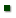Skip to main content
ARS Home » Pacific West Area » Pullman, Washington » Northwest Sustainable Agroecosystems Research » Research » Publications at this Location » Publication #182141

Title: ASSESSING THE USEFULNESS OF SIMPLE MATHEMATICAL MODELS TO DESCRIBE SOIL CARBON DYNAMICS.

AuthorKAMANIAN, ARMENMANORANJAN, VHuggins, DavidSTOCKLE, CLAUDIO

 Submitted to: USDA Symposium on Greenhouse Gases & Carbon Sequestration in Agriculture and Forestry Publication Type: Abstract Only Publication Acceptance Date: 1/1/2005 Publication Date: 4/1/2006 Citation: Assessing the Usefulness of Simple Mathematical Models to Describe Soil Carbon Dynamics. Kemanian, A.R., Manoranjan, V.S., Huggins, D.R., Stockle, C.O. Third USDA Symposium on Greenhouse Gases and Carbon Sequestration in Agriculture and Forestry. March 21-24, 2005, Baltimore, Maryland. http://soilcarboncenter.k-state.edu/conference/Technical_Sessions_Oral_Presentations.htm Interpretive Summary: Technical Abstract: The rate of C storage (Cs) in the soil depends on the balance between the inputs of C from organic residues (Ci) and the outputs due to microbial oxidation of organic matter. Due to the complexity of the processes affecting the input and output rates, analytical solutions to C dynamics are not feasible. Partial information regarding particular processes like residue decomposition and C turnover, together with the effect of environmental factors on microbial activity, have been compiled and encapsulated in so-called mechanistic models of soil organic matter dynamics. The use of these models has been promoted under the assumption that complex interactions among several factors are accounted for quantitatively. It must be noted, however, that the structure of these mechanistic models is relatively simple. We hypothesize that simple mathematical models could be equally or more effective at representing soil carbon dynamics. We present empirical models of soil C dynamics of the general form dCs/dt = h(Cs)Ci + k(Cs)Cs, where h and k are the residue humification and apparent soil C decomposition coefficients. The simplest solution to this equation results from assuming that h and k are constant, which implies that under steady state Cs = hCi/k. This implies that the storage capacity of the soil is linearly dependent on Ci. There is evidence, however, that soils have a finite capacity to store C. In addition, the Cs constituents have different turnover rates i.e. k is a weighted average of the individual k's of each soil C fraction. We know that as Cs changes k does not remain constant, as each individual C constituent changes at different rates. We explore the behavior of models in which h and k are functions of Cs. The general behavior of these models in response to changes in CI and Cs is presented. When possible, some models were parameterized based on observed values of soils at steady state and a small set of assumptions. The analysis indicates that making h a function Cs provides a simple way of representing a "Cs carrying capacity". Making k a function of Cs provides a means for accelerating the turnover rate as Cs increases, with the underlying assumption that the higher the Cs content the lower the soil recalcitrant C fraction. These simple models could be useful to predict Cs dynamics and also provide feedback information to improve existing mechanistic models.